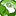# New to Qlik Sense

If you’re new to Qlik Sense, start with this Discussion Board and get up-to-speed quickly.

Announcements
Welcome to Qlik Community! Check out our new navigation! FIND OUT MORE
cancel
Showing results for
Did you mean:Contributor III

## Aggregating data without losing granularity

I'm struggling to figure out how to aggregate data without it causing me to not be able to measure by other dimensions.

Key Dimensions

[Mon] = month

[Resource Group] = The dimension which connects [FTE] and [ResourceCost]

[Role] = a sub-dimension of [Resource Group]

[Sector] = A dimension for clients  - used in a master dimension, with drill down to [Database Code]

[Type] = Whether or not the [Resource Group] is shared across [Model] - for the purpose of this calculation we are looking at 'Mix'

Key Measures

[FTE] = Single values stored per ([Resource Group], [Role], [Mon]) - represents the number of resources

[ResourceCost] = Single values stored per ([Resource Group], [Mon]) - represents the cost of resources

What I'm trying to calculate

The master measure I'm looking to calculate is the [Resource Cost] for each [Mon] and [Role] (or at least [Mon] and [Resource Group].

Calculating this in Excel I would:

1. For each [Role] and [Mon], calculate the percentage of [FTE] of [Type] = 'Mix' and [Model] = 'Heritage' as a percentage of the total [FTE] in that [Resource Group]

2. Multiply each number by the [Resource Cost] in that ([Mon], [Resource Group]) to calculate the cost for each [Role] (or at least [Resource Group])

Attempts

1.

``(Sum({<[Model]={'Heritage'}>*<[Type]={'Mix'}>} ([FTE]*[ResourceCost])) / (Sum([FTE])``

or

``Sum({<[Model]={'Heritage'}>*<[Type]={'Mix'}>} [FTE])/(Sum(FTE/[ResourceCost])))``

Both of the above attempts evaluate correct values against single values of [Resource Group], however against multiple or all values of [Resource Group] (or drilled down to [Role] level) don't evaluate correctly - the intended result is the sum of the individually evaluated values against [Resource Group].

2.

``Sum(Aggr(Sum({<[Model]={'Heritage'}>*<[Type]={'Mix'}>} ([FTE]*[ResourceCost])) / (Sum([FTE])),[Resource Group]))``

or

``Sum(Aggr(Sum({<[Model]={'Heritage'}>*<[Type]={'Mix'}>} [FTE])/(Sum(FTE/[ResourceCost])),[Resource Group]))``

Aggregating either of these makes the calculation work correctly against [Resource Group] in all scenarios but then the aggregate means that the calculation means it won't evaluate against the other dimensions [Mon] and [Sector]

I'm at a bit of a loss on how to get this to evaluate correctly. Any ideas?

2 RepliesContributor III
Author
Anyone have any ideas?Partner - Master

Hi Thomas,

Can you share a sample QVF and an Excel file with the desired outcome?

Jordy

Climber

Work smarter, not harderTags
Community Browser